# php?去掉空格

``````
[content_sc] =>

\$row['content_tc'] = str_replace('	', '', \$row['content_tc']);

[content_sc] =>

``````

...全文
198 点赞 收藏 17

17 条回复

``````foreach (\$information as \$row) {
\$row['content_tc']=preg_replace("/[ \t]+/", '',\$row['content_tc']);
\$row['content_tc']=nl2br(\$row['content_tc']);
\$informationAfterHandle[] = \$row;
}
``````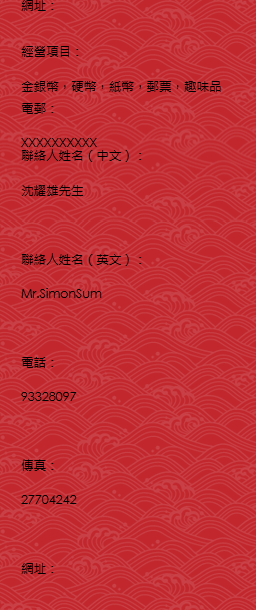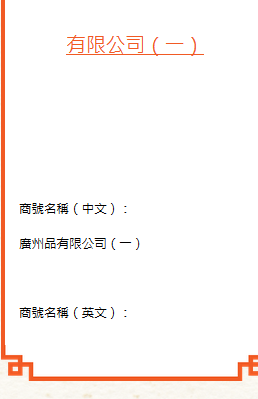xuzuning 2015-10-23
``````foreach (\$information as \$row) {
\$row['content_tc']=preg_replace("/[ \t]+/", '',\$row['content_tc']);
\$row['content_tc']=nl2br(\$row['content_tc']);
\$informationAfterHandle[] = \$row;
}
``````

``````
foreach (\$information as \$row) {
\$row['content_tc']=preg_replace("/\r\n/", '<br/>',\$row['content_tc']);
\$row['content_tc']=preg_replace("/[ \t]+/", '',\$row['content_tc']);
}
\$informationAfterHandle[] = \$row;
``````

``````
foreach (\$information as \$row) {
\$row['content_tc']=preg_replace("/[ \t]+/", '',\$row['content_tc']);
\$row['content_tc']=preg_replace("/\r\n/", '<br/>',\$row['content_tc']);
}
\$informationAfterHandle[] = \$row;

``````

xuzuning 2015-10-23

xuzuning 2015-10-23

``````
\$row['content_tc']=preg_replace("/\r\n/", '<br/>',\$row['content_tc']);
``````

xuzuning 2015-10-23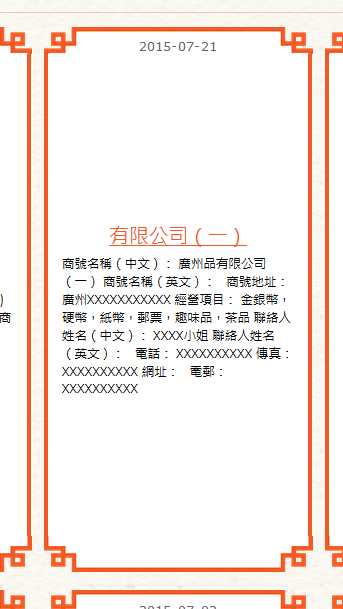\$row['content_tc'] = preg_replace("/[ \t]+/", '', \$row['content_tc']); 换行没了

xuzuning 2015-10-23
\$row['content_tc'] = preg_replace("/[ \t]+/", '', \$row['content_tc']); 改错都改傻了吧？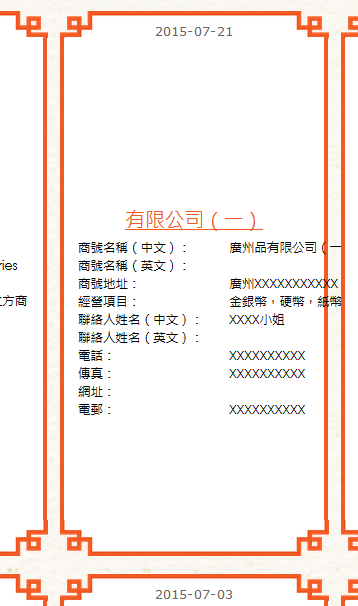preg_replace("/[ \t]+/", '', \$row['content_tc']);加上这句，显示效果和原来一样

xuzuning 2015-10-23
html 方式下当然不会换行！ 你在浏览器中 查看源代码 就知道了

xuzuning 2015-10-23
html 方式下当然不会换行！

``````
<?php
\$a="1 2  3   45
6  789";
echo (str_replace('    ', '', \$a));
?>
``````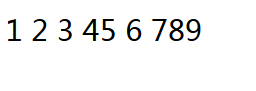``````
<html>
<meta charset="utf-8">
<body>
<?php
\$s =<<< TXT

TXT;
echo preg_replace("/[ \t]+/", '', \$s);
?>
</body>
</html>``````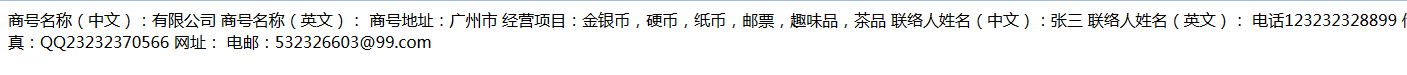xuzuning 2015-10-23
``````\$s =<<< TXT

TXT;
echo preg_replace("/[ \t]+/", '', \$s);``````
``````商号名称（中文）：有限公司

``````

1.9w+

“超文本预处理器”，是在服务器端执行的脚本语言，尤其适用于Web开发并可嵌入HTML中。PHP语法利用了C、Java和Perl，该语言的主要目标是允许web开发人员快速编写动态网页。